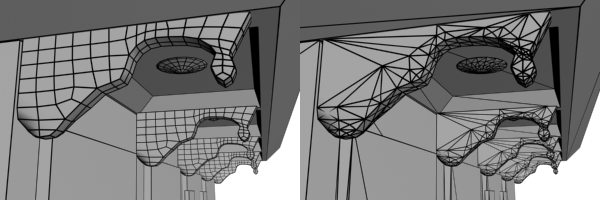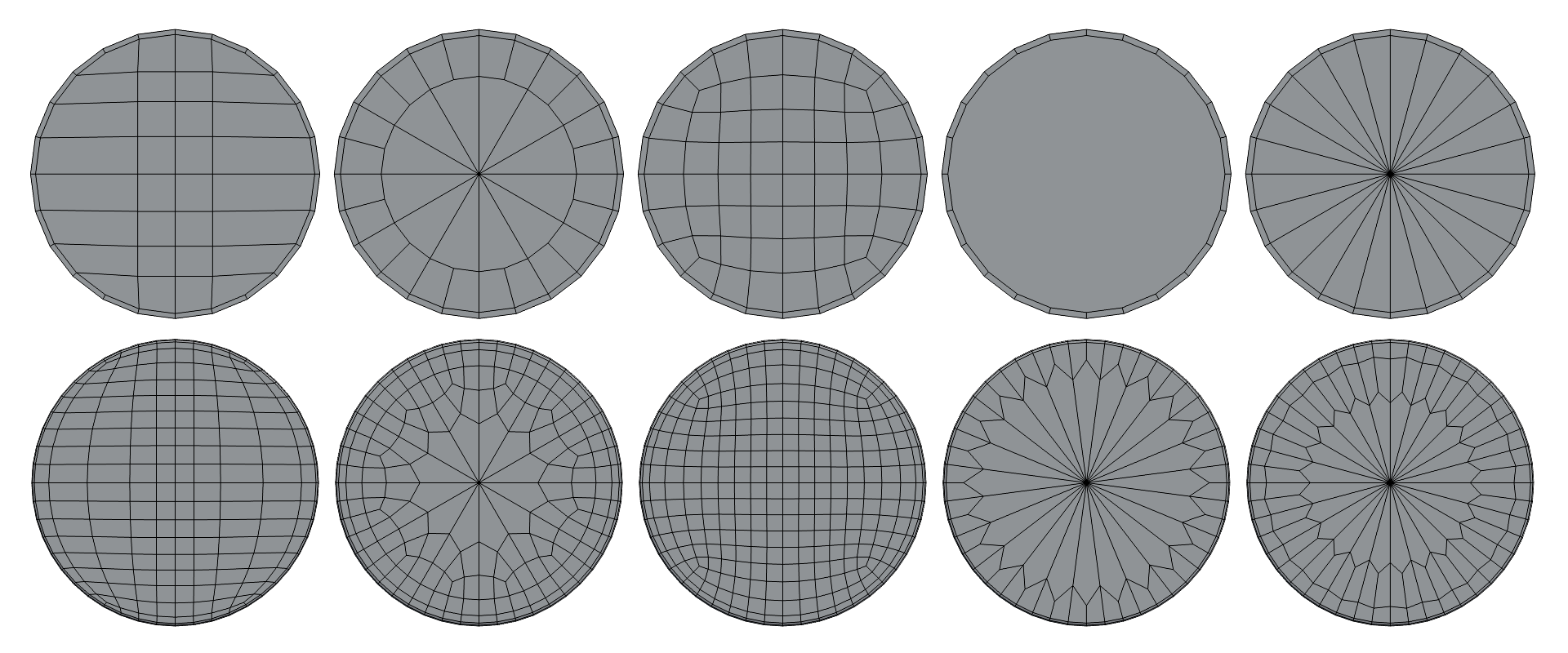# 3D Topology

a year ago

3D topology is a branch of mathematics that deals with the study of shapes and their properties. In the context of 3D modeling and computer graphics, topology refers to the way that the vertices, edges, and faces of a 3D model are connected to each other.

There are a few key concepts that are important to understand when it comes to 3D topology. One of these is the idea of a topological mesh, which is a 3D model made up of a set of interconnected polygons. These polygons are typically triangular or quadrilateral in shape, and they are connected to each other at their edges.
The way that the polygons in a topological mesh are connected to each other is called the topology of the mesh. The topology of a mesh is important because it determines how the mesh will deform when it is animated or otherwise transformed. A well-designed topology will allow the mesh to deform smoothly and naturally, while a poorly designed topology can cause the mesh to stretch or distort in unnatural ways.There are a few key factors that can impact the topology of a 3D model. One of these is the number of polygons in the model. A model with more polygons will generally have a more detailed and accurate topology, but it will also be more computationally expensive to render. As a result, 3D artists often need to balance the level of detail in their models with the performance requirements of their projects.

Another factor that can impact topology is the distribution of polygons on the 3D model. A model with a uniform distribution of polygons will generally deform more smoothly than a model with a non-uniform distribution. This is because the vertices of the polygons in a uniformly distributed model will move more predictably when the model is deformed.
There are a few different techniques that 3D artists can use to optimize the topology of their models. One of these is the use of edge loops, which are groups of edges that follow the contours of the model. Edge loops help to define the shape of a model and make it easier to control the flow of the topology.

Another technique is the use of edge splits, which are used to add additional vertices to the model. This can be useful for adding detail to certain areas of the model, such as around the eyes or mouth, where the topology needs to be more accurate to capture the finer details of the shape.Overall, 3D topology is an important consideration for any 3D artist. By understanding the principles of topology and using the right techniques to optimize the topology of their models, artists can create more realistic and believable 3D characters and environments.

Keep in touch:)